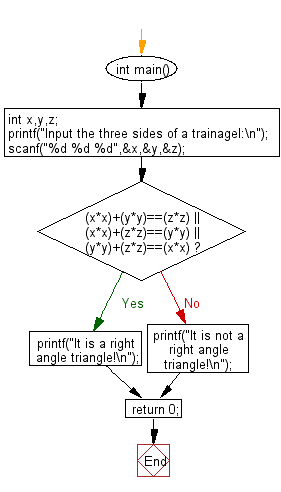﻿ C : Check if three sides of a triangle form a right triangle

# C Exercises: Check three sides of a triangle form a right triangle or not

## C Basic Declarations and Expressions: Exercise-134 with Solution

Write a C program to check whether the three given lengths (integers) of three sides of a triangle form a right triangle or not. Print "Yes" if the given sides form a right triangle otherwise print "No".

Input:
Integers separated by a single space.
1 <= length of the side <= 1,000

Sample Solution:

C Code:

``````#include<stdio.h>

int main()
{
int x, y, z;

// Prompt the user to input the three sides of a triangle
printf("Input the three sides of a triangle:\n");

// Read the input values for x, y, and z
scanf("%d %d %d", &x, &y, &z);

// Check if the triangle is a right angle triangle
if ((x*x) + (y*y) == (z*z) || (x*x) + (z*z) == (y*y) || (y*y) + (z*z) == (x*x))
{
// If the condition is true, print that it's a right angle triangle
printf("It is a right angle triangle!\n");
}
else
{
// If the condition is false, print that it's not a right angle triangle
printf("It is not a right angle triangle!\n");
}

return 0; // End of the program
}
``````

Sample Output:

```Input the three sides of a trainagel:
12
11
13
It is not a right angle triangle!
```

Flowchart:C programming Code Editor:

What is the difficulty level of this exercise?

Test your Programming skills with w3resource's quiz.

﻿